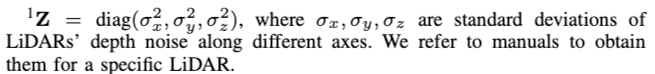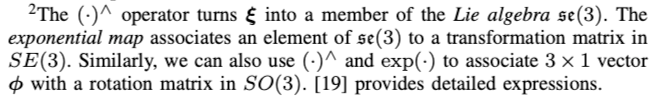# 0. 简介

[video(video-1K0JxXXg-1664086516393)(type-bilibili)(url-https://player.bilibili.com/player.html?aid=756354450)(image-https://img-blog.csdnimg.cn/img_convert/6d80b88ca2bd11a81cf74c03ac866921.jpeg)(title-[港科大] M-LOAM: 多激光雷达SLAM和标定)]

# 1. 文章贡献

1. 通过自动初始化，计算所有关键状态，包括连续帧之间的运动以及后续阶段的外参。它可以在任意位置开始，而无需任何先前的机械配置或校准对象知识
2. 使用常规收敛准则的在线自标定与里程计同时执行，它具有以完全无监督的方式监控收敛性和触发终止的能力
3. 基于滑动窗口的里程计，充分利用来自多个 LiDAR 的信息，该实现可以解释为小规模的帧到地图的匹配，这进一步减少了连续帧到帧的帧累积的漂移
4. 使用二阶段方法进行建图，捕获传感器噪声并传播不确定性，来消除姿势估计和外参估计的外部扰动，这种方法使建图过程能够了解不确定性，并有助于我们保持全局地图的一致性以及提高系统的鲁棒性以进行长期导航任务。

# 2. 先验知识

$\mathbf{T}=\left[\begin{array}{cc} \mathbf{R} & \mathbf{p} \\ \mathbf{0}^{\top} & 1 \end{array}\right]$

### 2.1 最大似然估计

$\hat{\mathbf{x}}_{k}=\underset{\mathbf{x}_{k}}{\arg \max } p\left(\mathcal{F}_{k} \mid \mathbf{x}_{k}\right)=\underset{\mathbf{x}_{k}}{\arg \min } f\left(\mathbf{x}_{\mathbf{k}}, \mathcal{F}_{k}\right)$

• $\mathcal{F}_{k}$ 表示第k帧的有效特征
• $\mathbf{x}_{k}$ 表示待优化的状态
• $f(\cdot)$ 表示目标函数

$\hat{\mathbf{x}}_{k}=\underset{\mathbf{x}_{k}}{\arg \min } \sum_{i=1}^{m} \rho\left(\left\|\mathbf{r}\left(\mathbf{x}_{k}, \mathbf{p}_{k i}\right)\right\|_{\mathbf{\Sigma}_{i}}^{2}\right)$

• $\rho(\cdot)$ 表示鲁棒性Huber损失，用于处理outlier
• $\mathbf{r}(\cdot)$ 表示残差函数
• $\Sigma_i$ 表示协方差矩阵

## 不确定性表示

$\mathbf{p}=\overline{\mathbf{p}}+\boldsymbol{\zeta}, \quad \boldsymbol{\zeta} \sim \mathcal{N}(\mathbf{0}, \mathbf{Z})$

• $\overline{\mathbf{p}}$ 表示不含噪声的点
• $\zeta \in \mathbb{R}^{3}$ 是零均值的高斯扰动变量， $\mathbf{Z}$ 是激光测量的噪声协方差
• 为了使得式（3）与转换矩阵(i.e., $\left.\mathbf{p}_{h}^{\prime}=\mathbf{T} \mathbf{p}_{h}\right)$ 更加紧凑，我们使用齐次坐标系来表示：

$\mathbf{p}_{h}=\left[\begin{array}{l} \overline{\mathbf{p}} \\ 1 \end{array}\right]+\mathbf{D} \boldsymbol{\zeta}=\overline{\mathbf{p}}_{h}+\mathbf{D} \boldsymbol{\zeta}, \quad \boldsymbol{\zeta} \sim \mathcal{N}(\mathbf{0}, \mathbf{Z})$

• $\mathbf{D}$ 是将3x1向量转换为齐次坐标的矩阵

LIDARS深度测量误差（也称为传感器噪声）主要受目标距离的影响，矩阵 $\mathbf{Z}$ 被简单的设置为常值矩阵。$\mathbf{T}=\exp \left(\boldsymbol{\xi}^{\wedge}\right) \overline{\mathbf{T}}, \quad \boldsymbol{\xi} \sim \mathcal{N}(\mathbf{0}, \boldsymbol{\Xi})$

• $\overline{\mathbf{T}}$ 是不含噪声的变换矩阵
• $\xi \in \mathbb{R}^{6}$ 是协方差为 $\Xi$ 的小扰动变量• Degenerate Pose Estimatio，例如在受限制的环境中缺乏几何结构，它通常在其退化方向上不确定性。
• Extrinsic Perturbation，该项始终存在，由于外参误差的存在。这种扰动会对多传感器系统的测量精度造成不良影响,但很难测量。

# 3. 主要工作---在线标定

1. 激光雷达是同步的，这意味着不同激光雷达之间的时间延迟几乎为零。
2. 在校准初始化期间，平台经历充分的旋转和平移运动。
3. 主激光雷达的局部地图应与辅助激光雷达共享一个重叠的视场，以便在精细化过程中进行特征匹配，以缩短标定阶段。这可以通过移动机器人来实现。

### 3.1 外参粗校准（Calibration of Multi-LiDAR System）

$\mathbf{R}_{l_{k}^{i}}^{l_{k-1}^{i}} \mathbf{R}_{l^{i}}^{b}=\mathbf{R}_{l^{i}}^{b} \mathbf{R}_{b_{k}}^{b_{k-1}}$

$\left(\mathbf{R}_{l_{k}^{i}}^{l_{k-1}^{i}}-\mathbf{I}_{3}\right) \mathbf{t}_{l^{i}}^{b}=\mathbf{R}_{l^{i}}^{b} \mathbf{t}_{b_{k}}^{b_{k-1}}-\mathbf{t}_{l_{k}^{i}}^{l_{k-1}^{i}}$

M-LOAM这里通过堆叠外参中旋转的约束，构建 $AX=0$ 的等式，通过对矩阵 $A$ 的SVD分解来获得外参中的旋转部分。当求得旋转之后，通过堆叠外参中的平移约束可以构建与求解最小二乘问题，即可求得位移。

### 3.2 外参细标定

1. 首先构建Pr LiDAR的Local map，方法是将滑窗内点云都合并到Pivot帧（Pivot定义见优化变量节）的坐标系下。

2. 随后为优化问题添加特征关联因子。

• 对于滑窗内每个不是Fixed的帧的Pr LiDAR的点云的特征点（Edge point 或Planer point)，将其直接通过该帧的初始Pose与Pr LiDAR的Local Map关联（K-d Tree)。则优化时会更新该帧的pose。
• 对于滑窗内每个不是Fixed的帧的Au LiDAR的点云的特征点（Edge point 或Planer point)，将其通过Pr LiDAR的初始Pose以及该Au LiDAR到Pr Lidar的外参，与Pr LiDAR的Local Map关联。则优化时不仅会更新该帧的Pr LiDAR的pose，还会更新该Au LiDAR与Pr LiDAR的外参。
3. 执行优化。

\begin{aligned} \mathcal{X} &=[\mathcal{X}_{f},\qquad \mathcal{X}_{v}, \qquad \qquad \qquad \mathcal{X}_{e}] \\ &= [\mathbf{x}_{1}, \cdots, \mathbf{x}_{p}, \mathbf{x}_{p+1}, \cdots, \mathbf{x}_{N+1}, \mathbf{x}_{l^{2}}^{b}, \cdots, \mathbf{x}_{l^{l}}^{b}] \end{aligned}

\begin{aligned} \mathbf{x}_{k} &=\left[\mathbf{t}_{b_{k}}^{w}, \mathbf{q}_{b_{k}}^{w}\right], \quad k \in[1, N+1] \\ \mathbf{x}_{l^{i}}^{b} &=\left[\mathbf{t}_{l^{i}}^{b}, \mathbf{q}_{l^{i}}^{b}\right], \quad i \in[1, I], \end{aligned}

• $\mathbf{x}_k$ 是主激光雷达在世界坐标系下不同时间戳的状态
• $\mathbf{x}_{l^{i}}^{b}$ 表示主激光雷达到第i辅助激光雷达的外参
• $N+1$ 是滑动窗口中的状态数量

### 3.3 外参收敛性判断

1. 在第4行，我们从cost function的信息矩阵中计算 $\lambda$
2. 在5-7行，如果 $\lambda$ 大于阈值，则对外参进行更新。
3. 在第8行，我们使用的外参候选值来检查收敛性。
4. 在第9-10行，收敛性判断条件满足，因此触发停止标定。然后计算 $\mathcal{L}$ 的采样均值，作为输出的外参结果，并且采样协方差作为标定的协方差。

# 4. 参考链接

https://blog.csdn.net/u013019296/article/details/117538703

https://jishuin.proginn.com/p/763bfbd785e3

http://epsilonjohn.club/2022/05/23/M_LOAM%E8%AE%BA%E6%96%87%E9%98%85%E8%AF%BB/